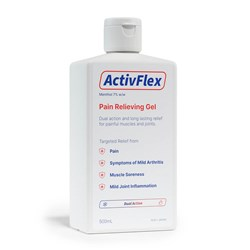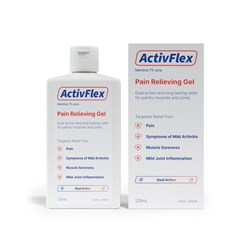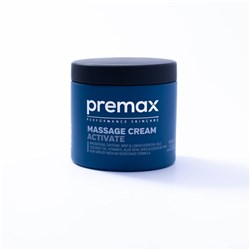# Pain Relief Creams and Gels

Grid List\$37.99
Increase value Decrease value\$24.99
Increase value Decrease value
\$29.99
Increase value Decrease value
\$29.99
Increase value Decrease value
\$24.95
Increase value Decrease value
\$29.00
Increase value Decrease value
\$20.50
Increase value Decrease value
\$79.90
Increase value Decrease value
\$16.17
Increase value Decrease value
Tiger Balm White 18g
\$16.63
Increase value Decrease value
\$58.01
Increase value Decrease value
\$26.20
Increase value Decrease value
\$13.23
Increase value Decrease value
\$24.85
Increase value Decrease value
\$48.69
Increase value Decrease value
\$28.25
Increase value Decrease value
\$9.20
Increase value Decrease value
\$21.10
Increase value Decrease value\$39.00
Increase value Decrease value
\$29.99
Increase value Decrease value
\$39.00
Increase value Decrease value
\$39.00
Increase value Decrease value
\$39.00
Increase value Decrease value
\$38.50
Increase value Decrease value
\$11.00
Increase value Decrease value
\$16.34
Increase value Decrease value
\$19.21
Increase value Decrease value
Metsal 50g
In Stock
\$15.30
Increase value Decrease value
Metsal 125g
In Stock
\$22.65
Increase value Decrease value
Metsal 500g
In Stock
\$45.22
Increase value Decrease value
Mentholatum Ice Gel 100g
\$12.17
Increase value Decrease value
\$40.00
Increase value Decrease value
Grid List
##### Products to compare:
Comparing Products